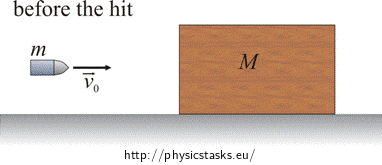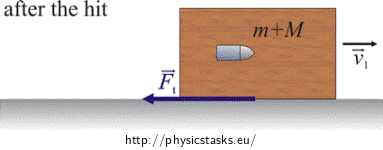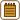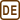## A block struck by a bullet

A wooden block with mass M = 3 kg is lying on a horizontal table. It is hit by a bullet with mass m = 5 g which moves horizontally. The bullet remains in the block after colliding with it. The block moves on the table a distance d = 25 cm. The coefficient of kinetic friction μk = 0.2. Find the starting speed of the bullet.

• #### Analysis

To solve the problem we use the law of conservation of linear momentum, by which we determine the speed of the block after it is hit by the bullet, and the law of conservation of energy.

• #### Hint 1 – the law of conservation of linear momentum

Draw a picture of the situation. Express the momentum of the system of the bullet and the block just before the bullet hits the block and immediately after the hit.

• #### Hint 2 - the law of conservation of energy

How does the kinetic energy of the bullet change when hitting the block?

• #### Hint 3 – Stopping of the block

Why does the block (which moves after the hit) stop after some time? Into what does its kinetic energy change?

• #### Numerical calculation

We know:

$m=5\,\mathrm{g}=0.005\,\mathrm{kg}$ $M=3\,\mathrm{kg}$ $g=9.81\,\mathrm{m\cdot s^{-2}}$ $d=25\,\mathrm{cm}=0.25\,\mathrm{m}$ $\mu_k=0.2$

We want to determine:

$v_0=?$ $v_0=\frac{m+M}{m}\sqrt{2gd\mu_k}$ $v_0=\frac{0.005+3}{0.005}\sqrt{2{\cdot}9.81{\cdot}0.25{\cdot}0.2}\,\mathrm{m\cdot s^{-1}}$ $v_0=595\,\mathrm{m\cdot s^{-1}}$

The bullet before hitting the block moves with the speed $v_0=\frac{m+M}{m}\sqrt{2gd\mu_{k}}=595\,\mathrm{m\cdot s^{-1}}$ .

• #### COMPLETE SOLUTION

To solve the problem we use the law of conservation of linear momentum, by which we determine the speed of the block after it is hit by the bullet, and the law of conservation of energy.According to the law of conservation of linear momentum the momentum of the bullet and the block before the hit will be equal to the momentum of the block with the bullet after the hit.

The law of conservation of linear momentum: $$\qquad \vec{p_0}+ \vec{p_b}=\vec{p_1}$$

$$p_0$$...... the vector momentum of the bullet before the hit

$$p_b$$...... the vector momentum of the block before the hit

$$p_1$$...... the vector momentum of the system of the bullet and the block after the hit

$m\vec{v_0}+ 0=(m+M)\vec{v_1}$

$$v_0$$..... the vector velocity of the bullet before the hit

$$v_1$$..... the vector velocity of the system of the bullet and the block after the hit

We can rewrite the law of conservation of linear momentum in scalar form because the bullet and the block move along one line.

$mv_0=(m+M)v_1$

From this we express the speed v1:

$v_1=\frac{mv_0}{m+M}\tag{1}$

Part of the kinetic energy of the bullet changes to the kinetic energy of the block which is moving after the hit. Another part of the kinetic energy of the bullet changes to the internal energy of the bullet and block which warm up after the hit. We can write this down into an equation.

$E_{k0}=E_k+\Delta U$

$$E_{k0}$$...... the kinetic energy of the bullet before the hit

$$E_k$$........ the kinetic energy of the system of the bullet and the block immediately after the hit

$$\Delta U$$....... the change in the internal energy of the bullet and the block

$\frac{1}{2}mv_0^2=\frac{1}{2}(M+m)v_1^2+\Delta U$

The block stops due to the influence of the friction force. When stopping the block the friction force performs the work, the block and the table warm up and their internal energy increases. The kinetic energy of the block with the bullet (which they have immediately after the hit) equals the work which the friction force performs along the length of the stopping. We write this down into an equation.

$E_k=W$

$$E_k$$..... the kinetic energy of the system of the bullet and the block immediately after the bullet collides with the block

$$W$$...... the work of the friction force

$\frac{1}{2}(m+M)v_1^2=F_td\tag{2}$

The friction force is directly proportional to the normal force by which the block with the bullet acts upon the table:

$F_t = N\mu_k = (M+m)g\mu_k$

We substitute the friction force into equation (2):

$\frac{1}{2}(m+M)v_1^2=(M+m)g\mu_kd$

For v1 we substitute from equation (1):

$\frac{1}{2}(M+m)\frac{m^2v_0^2}{(M+m)^2}=(M+m)g\mu_kd$ $v_0^2=\frac{2(M+m)^2}{m^2}g\mu_kd$ $v_0=\frac{M+m}{m}\sqrt{2g\mu_kd}$ $v_0=\frac{0.005+3}{0.005}\sqrt{2{\cdot}9.81{\cdot}0.25{\cdot}0.2}\,\mathrm{m\cdot s^{-1}}$ $v_0=595\,\mathrm{m\cdot s^{-1}}$

Answer: The bullet before hitting the block moves with the speed $v_0=595\,\mathrm{m\cdot s^{-1}}$ .